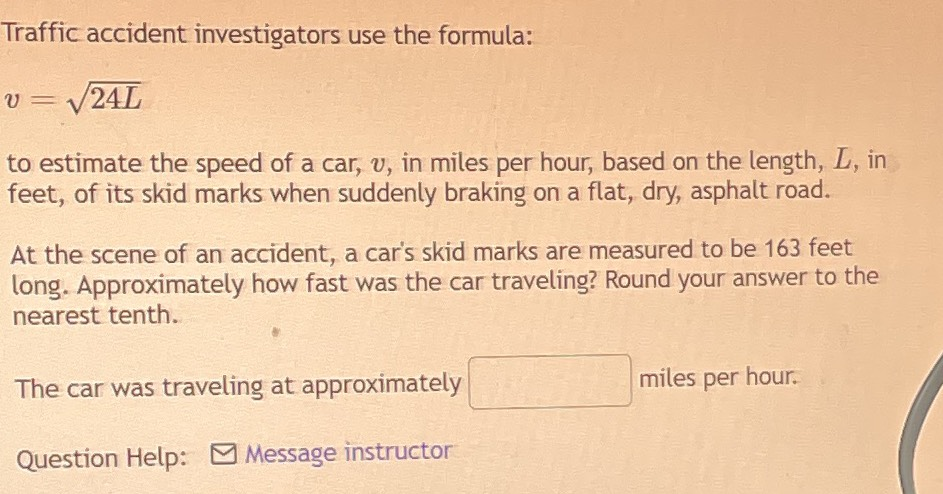### Still have math questions?

Algebra
QuestionTraffic accident investigators use the formula:

$$v = \sqrt { 24 L }$$

to estimate the speed of a car, $$v$$ , in miles per hour, based on the length, $$L$$ , in feet, of its skid marks when suddenly braking on a flat, dry, asphalt road.

At the scene of an accident, a car's skid marks are measured to be $$163$$ feet long. Approximately how fast was the car traveling? Round your answer to the nearest tenth.

The car was traveling at approximately _____miles per hour